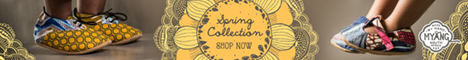# Gr. 8 Rainbow Mathematics Book 1

Gr. 8 Rainbow Maths Book 1

## IndexGr. 8 Rainbow Maths Book 1 Index
Doing calculations
Multiples and factors
Exponents
Integers
Common fractions
Percentages and decimal fractions
Input and output
Algebraic expressions and equations
Graphs
Financial mathematics
Geometric figures
Transformations
Geometry
Perimeter and area
Volume and surface area
Data

Natural numbers, whole numbers and integers
Commutative, associative and distributive properties
Factors, prime factors and factorising
Multiples and the lowest common multiple
Highest common factor and lowest common multiple of three-digit numbers
Finances – profit, loss and discount
Finances – Budget
Finances – loans and interest
Finances – Hire Purchase
Finances – exchange rates
Sequences that involve integers
Calculations with multiple operations
Properties of numbers and integers
Square numbers, cube numbers and more exponents
Square numbers and square roots

Representing square roots
Cube numbers and roots
Representing cube roots
Scientific notation
Laws of exponents: xm xn = xmn
Law of exponents: xm ÷ xn = xm − n
More laws of exponents: (xm)n = xmn
Law of exponents: (x°) = 1
Calculations with exponents
Calculations with multiple operations (square and cube numbers, square and cube roots)
Numeric patterns
Input and output values
Algebraic vocabulary
Like terms: whole numbers
Like terms: integers
Writing number sentences

Set up algebraic equations
Balance an equation
Substitution
Algebraic equations
Solving problems
Divide monomials, binomials and trinomials by integers or monomials
Simplify algebraic expressions
Calculate the square numbers, cube numbers and square roots of single algebraic terms
Multiple operations: rational numbers
Multiple operations
Division operations
Constructing geometric figures
Construction with a protractor
Parallel and perpendicular lines
Construct angles and a triangle
The sum of the interior angles of any triangle equals 180°

Constructing polygons
Polygons
Similar Triangles
Congruent triangles
Similar triangles problems